# Less Than Sign

Here we will learn about the less than sign including the symbol used to represent it, other comparison symbols and comparing numbers and expressions using the less than sign.

There are also inequality worksheets based on Edexcel, AQA and OCR exam questions, along with further guidance on where to go next if you’re still stuck.

## What is the less than sign?

The less than sign is a mathematical symbol used to compare numbers and expressions. Otherwise known as a comparison symbol.

The less than sign is

The wide end of the symbol always faces the bigger number or expression – the symbol looks open towards the bigger number and ‘points’ at the smaller value like an arrow.

For example,

This is read as ‘4 is less than 9’.

11<18 is read as ‘11 is less than 18’.

1.3<1.7 is read as ‘1.3 is less than 1.7’.

The less than sign is also used to represent inequalities in maths.

For example, x<3   is read as ‘x is less than 3’.

Step-by-step guide: Inequalities

There are more comparison symbols (or inequality symbols) you need to know.

• Equal to = using the equal symbol denotes when two values are exactly equal.

For example, 3+4=7.

• Not equal to this not equal sign means two values are definitely not equal.

For example, 3+4 10.

• Identical to \equiv symbol means two values are identical, even though they may be written in different forms.

For example, 4(x+2)\equiv 4x+8.

• Greater than > denotes when one value is bigger than another.

For example, 8>7.

Step-by-step guide: Greater than sign (coming soon)

• Less than or equal to \le denotes when we know a value is smaller or equal to.

• Greater than or equal to \ge denotes when we know a value is bigger or equal to.

### What is the less than sign?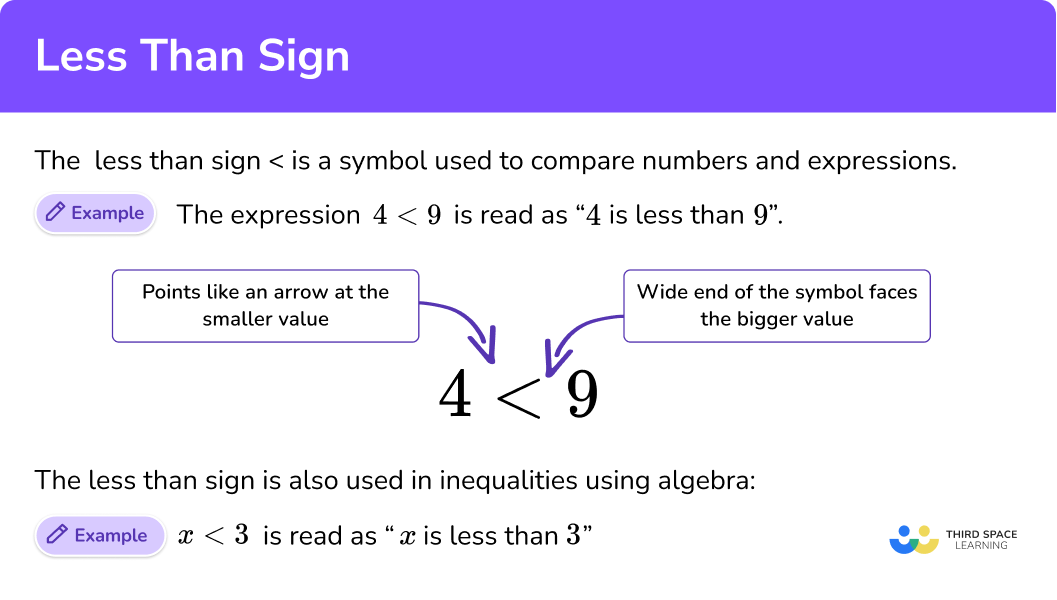## How to compare values using the less than sign

In order to compare values using the less than sign:

1. Compare the values given.
2. If less than, write the smaller value on the left hand side.
3. If less than, write the bigger value on the right hand side.
4. Write the values with the correct symbol, or place the numbers on the correct sides of a given symbol.

### Explain how to compare values using the less than sign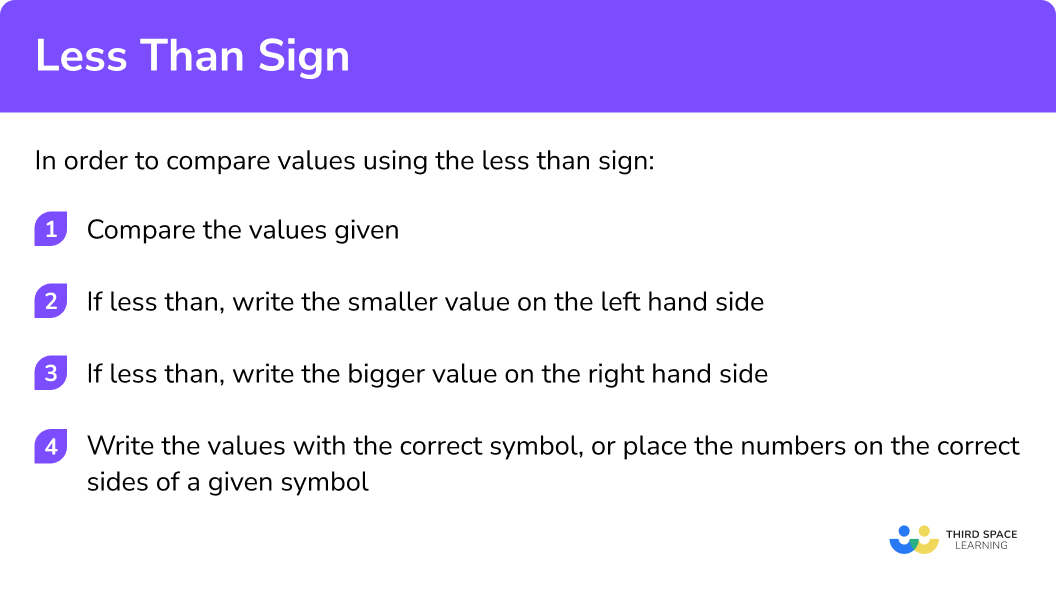## Related lessons on inequalities

Less than sign is part of our series of lessons to support revision on inequalities. You may find it helpful to start with the main inequalities lesson for a summary of what to expect, or use the step by step guides below for further detail on individual topics. Other lessons in this series include:

## Less than sign examples

### Example 1: comparing numbers

Write the correct sign, > or < , in the box.

1. Compare the values given.

Both values are negative numbers.

-28 is further away from zero, so it is the smaller of the two values.

2If less than, write the smaller value on the left hand side.

-28 is the smaller number, and is written on the left side, so the less than symbol is pointing to it like an arrow.

3If less than, write the bigger value on the right hand side.

-20 is the greater number, and is written on the right side, so we draw the less than symbol with the open end next to the larger value.

4Write the values with the correct symbol, or place the numbers on the correct sides of a given symbol.

### Example 2: comparing numbers

Write the correct sign, > or < , in the box.

Compare the values given.

If less than, write the smaller value on the left hand side.

If less than, write the bigger value on the right hand side.

Write the values with the correct symbol, or place the numbers on the correct sides of a given symbol.

### Example 3: comparing fractions

Which is smaller, \frac{3}{8} or \frac{4}{9}?

Compare the values given.

If less than, write the smaller value on the left hand side.

If less than, write the bigger value on the right hand side.

Write the values with the correct symbol, or place the numbers on the correct sides of a given symbol.

### Example 4: comparing fractions

Which is smaller, 3 \frac{4}{7} or 3 \frac{4}{9}?

Compare the values given.

If less than, write the smaller value on the left hand side.

If less than, write the bigger value on the right hand side.

Write the values with the correct symbol, or place the numbers on the correct sides of a given symbol.

### Example 5: comparing expressions

Which is smaller, 18 \times 9 or 186-35?

Compare the values given.

If less than, write the smaller value on the left hand side.

If less than, write the bigger value on the right hand side.

Write the values with the correct symbol, or place the numbers on the correct sides of a given symbol.

### Example 6: comparing expressions

Which is smaller, 5(3x+8) or 3(5x+8)?

Compare the values given.

If less than, write the smaller value on the left hand side.

If less than, write the bigger value on the right hand side.

Write the values with the correct symbol, or place the numbers on the correct sides of a given symbol.

### Common misconceptions

• Confusing the less than and greater than symbols

The biggest mistake is writing the symbols the wrong way round. Remember, the sign should point at the smallest number like an arrow.

For example, 15>8, \ ‘15 is greater than 8’, or 8<15, ‘8 is less than 15’.

• Equivalent fractions

When comparing fractions, we have to convert them into equivalent fractions with common denominators, so we can compare the numerators.

### Practice less than sign questions

1. Which is smaller, \frac{4}{11} or \frac{4}{9}? Write your answer using the less than comparison symbol.

\frac{4}{11}>\frac{4}{9}\frac{4}{11}<\frac{4}{9}\frac{4}{9}>\frac{4}{11}\frac{4}{9}<\frac{4}{11}Convert the fractions to equivalents with a common denominator.

\frac{4}{11}=\frac{36}{99}

\frac{4}{9}=\frac{44}{99}

Comparing the numerators tells us that \ \frac{36}{99}<\frac{44}{99} \ so \ \frac{4}{11}<\frac{4}{9}.

2. Which is smaller, -10 or -18? Write your answer using the less than comparison symbol.

-10>-18-10<-18-18>-10-18<-10Both values are negative. -18 is further from zero than -10, so -18 is smaller than -10.

3. Which is smaller, 2 \frac{6}{7} or 2 \frac{4}{5}? Write your answer using the less than comparison symbol.

2 \frac{6}{7}<2 \frac{4}{5}2 \frac{6}{7}>2 \frac{4}{5}2 \frac{4}{5}>2 \frac{6}{7}2 \frac{4}{5}<2 \frac{6}{7}Convert the fractions to equivalents with a common denominator.

2 \frac{6}{7}=\frac{100}{35}

2 \frac{4}{5}=\frac{98}{35}

Comparing the numerators tells us that \ \frac{98}{35}<\frac{100}{35} \ so \ 2 \frac{4}{5}<2 \frac{6}{7}

4. Which is smaller, 7 \times 16 or 15 \times 8? Write your answer using the less than comparison symbol.

7 \times 16>15 \times 87 \times 16<15 \times 815 \times 8<7 \times 1615 \times 8>7 \times 16Evaluating each expression gives,

7 \times 16=112

15 \times 8=120

So, 7 \times 16<15 \times 8.

5. Which is smaller, 20 \% of  3894 or 15 \% of 5686? Write your answer using the greater than comparison symbol.

20 \% of 3894<15 \% of 568620 \% of 3894>15 \% of 568615 \% of 5686<20 \% of 389415 \% of 5686>20 \% of 3894Evaluating each expression gives,

20 \% of 3894=778.8

15 \% of 5686=852.9

So, 20 \% of 3894<15 \% of 5686.

6. Which is smaller, 6(2 x+4) or 3(4 x+9)? Write your answer using the less than comparison symbol.

6(2x+4)>3(4x+9)6(2x+4)<3(4x+9)3(4x+9)>6(2x+4)3(4x+9)<6(2x+4)Evaluating each expression gives,

6(2x+4)=12x+24

3(4x+9)=12x+27

So, 6(2x+4)<3(4x+9).

### Less than sign GCSE questions

1. Write the correct sign <, =, or in each box.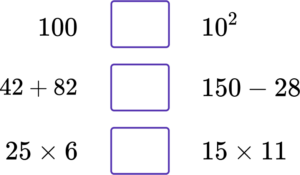(3 marks)

100=10^2

(1)

42+82 150-28

(1)

25\times 6 <15\times 11

(1)

2. Here are four number cards.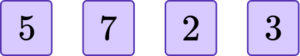Arrange the cards to give a possible expression below.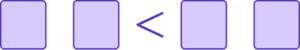(1 mark)

Any correct expression where the LHS is smaller than the RHS.

For example, 23<57 .

(1)

3. Here are two fractions.

Which fraction is smaller?

Complete the inequality expression below.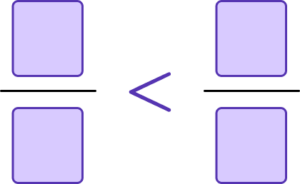(2 marks)

\frac{3}{4}=\frac{27}{36} \ or \ \frac{7}{9}=\frac{28}{36}

(1)

\frac{3}{4}<\frac{7}{8}

(1)

## Learning checklist

You have now learned how to:

• Recognise the less than sign
• Compare numbers and expressions
• Write expressions using the less than sign

## Still stuck?

Prepare your KS4 students for maths GCSEs success with Third Space Learning. Weekly online one to one GCSE maths revision lessons delivered by expert maths tutors.

Find out more about our GCSE maths tuition programme.